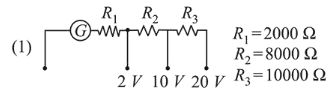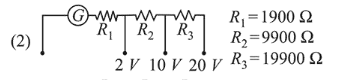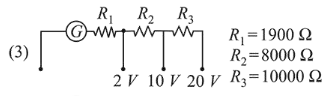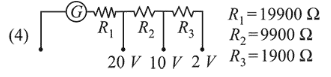# A galvanometer of resistanceQuestion:

A galvanometer of resistance $100 \Omega$ has 50 divisions on its scale and has sensitivity of $20 \mu \mathrm{A} /$ division. It is to be converted to a voltmeter with three ranges, of $0-2 \mathrm{~V}$, $0-10 \mathrm{~V}$ and $0-20 \mathrm{~V}$. The appropriate circuit to do so is :

1.2.3.4.Correct Option: , 3

Solution:

(3) $i_{g}=20 \times 50=1000 \mu A=1 \mathrm{~mA}$

Using, $V=i_{g}(G+R)$, we have

$2=10^{-3}\left(100+R_{1}\right)$

$R_{1}=1900 \Omega$

when, $V=10$ volt

$10=10^{-3}\left(100+R_{2}+R_{1}\right)$

$10000=\left(100+R_{2}+1900\right)$

$\therefore R_{2}=8000 \Omega$